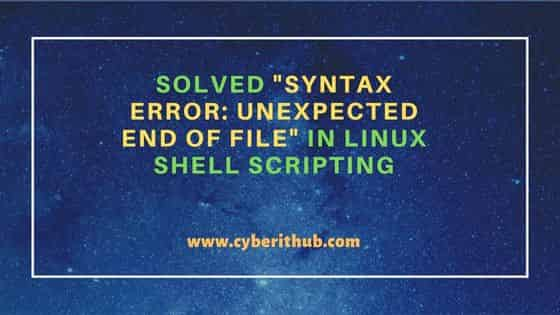# Solved "syntax error: unexpected end of File" in Linux Shell Scripting

In this article, we will see how to solve "syntax error: unexpected end of File" in Linux Shell Scripting. Last night when I was working on a simple shell script to calculate sum of two numbers, I noticed an error while running the script which was totally unexpected. Then I realized although the error is straight forward but it is so common that anyone can willingly or unwillingly can do this mistake. Then after I decided to write a short article about this explaining the different scenarios which can result in unexpected end of file error.## Solved "syntax error: unexpected end of File" in Linux Shell Scripting

The simple shell script which I was working is as shown below. Here I am calculating the sum of `a` and `b` values and storing it in variable `c`. Then finally displaying the output using `echo \$c` as you can see below.

```cyberithub@ubuntu:~\$ nano calculate
#! /bin/bash

a=5
b=7

sum()
{
c=\$((\$a+\$b))
echo \$c
}

sum()```

When I tried to run above script, it gave me below error output.

```cyberithub@ubuntu:~\$ ./calculate
./calculate: line 13: syntax error: unexpected end of file```

While unexpected end of file error can be encountered due to multiple reasons but most of the time it will be due to either parentheses or braces not opened or closed properly. Basically, the unexpected end of file error means you are trying to open something which was never closed as in bash of braces. If you need to know more about the error, then use `bash -x <script_name>`. Here `-x` switch is used for debugging purposes. This is more useful when you have a longer script where it is not at all easy to find errors.

Since in our case it's a pretty small script so we can easily identify the error. So if you see the output, it says the error is in `line 13` which is the below highlighted line.

```cyberithub@ubuntu:~\$ nano calculate
#! /bin/bash

a=5
b=7

sum()
{
c = \$((\$a+\$b))
echo \$c
}

sum()```

You might have identified the error by now. In `line 13`, opening and closing parentheses has been used to call the sum function which is incorrect. So if you remove the opening and closing parentheses like shown below and then try to run the script then you can see that sum function works properly.

```cyberithub@ubuntu:~\$ nano calculate
#! /bin/bash

a=5
b=7

sum()
{
c=\$((\$a+\$b))
echo \$c
}

sum```

Output

```cyberithub@ubuntu:~\$ ./calculate
12```

The other scenario you might encounter when you open the braces but forget to close it like in below example where you have opened the braces in below highlighted `line 12` but forgot or missed closing it. In that case also you will encounter `unexpected end of file` error when you try to run the script. Hence you need to be careful in closing braces.

```cyberithub@ubuntu:~\$ nano calculate
#! /bin/bash

a=5
b=7

sum()
{
c=\$((\$a+\$b))
echo \$c

sum```

Output

```cyberithub@ubuntu:~\$ ./calculate
./calculate: line 12: syntax error: unexpected end of file```

Hope the above explanation makes sense and helps you solve the error if you are also facing unexpected end of file error. Please let me know your feedback in the comment box !!

### 1 thought on “Solved "syntax error: unexpected end of File" in Linux Shell Scripting”

1.thanks for knowledge sharing